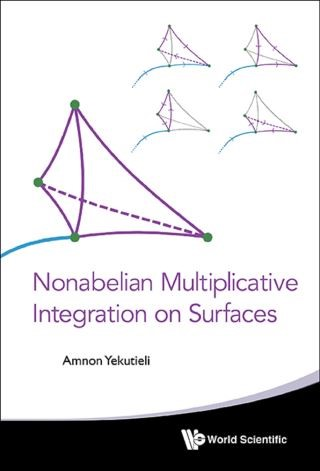## Nonabelian Multiplicative Integration On Surfaces• Description
• Author
• Info
• Reviews

### Description

Nonabelian multiplicative integration on curves is a classical theory. This volume is about the 2-dimensional case, which is much more difficult. In our construction, the setup is a Lie crossed module: there is a Lie group H, together with an action on it by another Lie group G. The multiplicative integral is an element of H, and it is the limit of Riemann products. Each Riemann product involves a fractal decomposition of the surface into kites (triangles with strings connecting them to the base point). There is a twisting of the integrand, that comes from a 1-dimensional multiplicative integral along the strings, with values in the group G.

The main result of this work is the 3-dimensional nonabelian Stokes theorem. This result is new; only a special case of it was predicted (without proof) in papers in mathematical physics. Our constructions and proofs are of a straightforward nature. There are plenty of illustrations to clarify the geometric constructions.

Our volume touches on some of the central issues (e.g., descent for nonabelian gerbes) in an unusually down-to-earth manner, involving analysis, differential geometry, combinatorics and Lie theory — instead of the 2-categories and 2-functors that other authors prefer.

Contents:
• Introduction
• Polyhedra and Piecewise Smooth Geometry
• Estimates for the Nonabelian Exponential Map
• Multiplicative Integration in Dimension One
• Multiplicative Integration in Dimension Two
• Quasi Crossed Modules and Additive Feedback
• Stokes Theorem in Dimension Two
• Square Puzzles
• Stokes Theorem in Dimension Three
• Multiplicative Integration on Triangular Kites